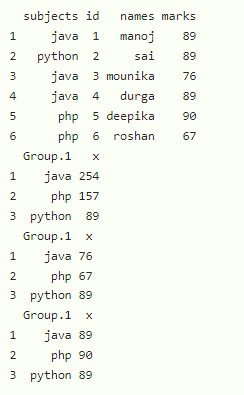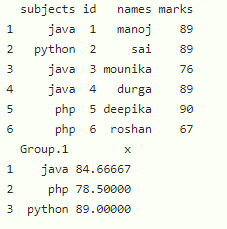GeeksforGeeks App
Open AppBrowser
Continue

# How to Use aggregate Function in R

In this article, we will discuss how to use aggregate function in R Programming Language.

aggregate() function is used to get the summary statistics of the data by group. The statistics include mean, min, sum. max etc.

Syntax:

aggregate(dataframe\$aggregate_column, list(dataframe\$group_column), FUN)

where

• dataframe is the input dataframe.
• aggregate_column is the column to be aggregated in the dataframe.
• group_column is the column to be grouped with FUN.
• FUN represents sum/mean/min/ max.

Example 1: R program to create with 4 columns and group with subjects and get the aggregates like minimum, sum, and maximum.

## R

 `# create a dataframe with 4 columns``data = ``data.frame``(subjects=``c``(``"java"``, ``"python"``, ``"java"``,``                             ``"java"``, ``"php"``, ``"php"``),``                  ``id=``c``(1, 2, 3, 4, 5, 6),``                  ``names=``c``(``"manoj"``, ``"sai"``, ``"mounika"``,``                          ``"durga"``, ``"deepika"``, ``"roshan"``),``                  ``marks=``c``(89, 89, 76, 89, 90, 67))`` ` `# display``print``(data)`` ` `# aggregate sum of marks with subjects``print``(``aggregate``(data\$marks, ``list``(data\$subjects), FUN=sum))`` ` `# aggregate minimum  of marks with subjects``print``(``aggregate``(data\$marks, ``list``(data\$subjects), FUN=min))`` ` `# aggregate maximum of marks with subjects``print``(``aggregate``(data\$marks, ``list``(data\$subjects), FUN=max))`

Output:Example 2: R program to create with 4 columns and group with subjects and get the average (mean).

## R

 `# create a dataframe with 4 columns``data = ``data.frame``(subjects=``c``(``"java"``, ``"python"``, ``"java"``,``                             ``"java"``, ``"php"``, ``"php"``),``                  ``id=``c``(1, 2, 3, 4, 5, 6),``                  ``names=``c``(``"manoj"``, ``"sai"``, ``"mounika"``,``                          ``"durga"``, ``"deepika"``, ``"roshan"``),``                  ``marks=``c``(89, 89, 76, 89, 90, 67))`` ` `# display``print``(data)`` ` `# aggregate average of marks with subjects``print``(``aggregate``(data\$marks, ``list``(data\$subjects), FUN=mean))`

Output:My Personal Notes arrow_drop_up